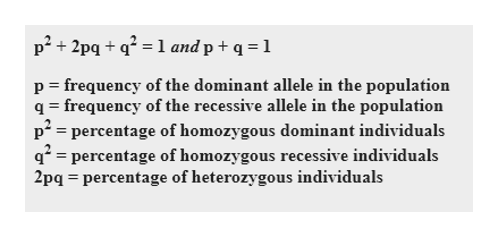# In our class of 130 students, 64 (49%) are unable to taste PTC.What is the percentage of individuals in the population that are tasters?What is the frequency of the dominant and recessive alleles?What percentage of the population are heterozygous for the trait?

Question

In our class of 130 students, 64 (49%) are unable to taste PTC.

1. What is the percentage of individuals in the population that are tasters?
2. What is the frequency of the dominant and recessive alleles?
3. What percentage of the population are heterozygous for the trait?
check_circle

Step 1

PTC tasting ability is a simple genetic trait that is dominant. It is governed by a pair of alleles, dominant T for tasting and recessive t for non-tasting.

Let us assume that the population is in Hardy Weinberg equilibrium. Equations are provided on the white board.

So, 49% is the frequency of the recessive phenotype. So, the frequency of tt is 49%, which means that q2 = 0.49 by definition. If q2 = 0.49, then q = 0.7.help_outlineImage Transcriptionclosep2+2pq 1 and p + q = 1 p frequency of the dominant allele in the population q frequency of the recessive allele in the population p percentage of homozygous dominant individuals qpercentage of homozygous recessive individuals 2pq percentage of heterozygous individuals fullscreen
Step 2

1. Given there are 130 students out of which 61 are non tasters. So, the number of taster individuals equals (130-61)= 66.

The percentage of individuals in the population that are tasters = 66/130 *100 = 50.7 % or 51%.

Hence, 51% of individuals are PTC tasters.

Step 3

2. Now, p is the frequency of dominant allele in the population and  q is the frequency of recessive allele in the population.

From step 1, value of q is 0.7.

We also know, p+q  = 1. So, value of p is (1 - 0.7)= 0....

### Want to see the full answer?

See Solution

#### Want to see this answer and more?

Solutions are written by subject experts who are available 24/7. Questions are typically answered within 1 hour.*

See Solution
*Response times may vary by subject and question.
Tagged in

### Biology